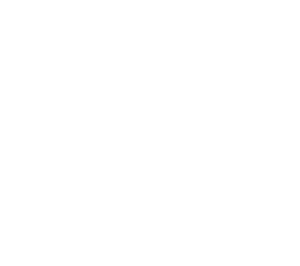I’ll start with a question – to how many significant figures is the number 500?

What does “significant” mean?
Consider the number 315 – which digit can you change which would have the least effect on the size of the number? Clearly the 5: increase or decrease it by 1, and 315 changes by 1. But move the 3 up or down 1, and the number will change by 100. So, the 3 is more “significant” than the 5 – and the further left a digit is, the more significant its value.

Why do we need to round numbers anyway?
Because, strange to say, numbers can be too accurate! What is the population of this town – answer: 15,263. But, hold on – someone has just been born; or someone has just died; or moved away, so the answer is no longer true. Better to say “about 15000.” Or consider this calculation: a rectangle has sides measured at 2.1cm and 5.3cm, so its area is 11.13cm2.  But since the dimensions were measurements, they weren’t accurate – the area could be as low as 2.05 × 5.25, or as high as 2.15 × 5.35, giving a range 10.7625 to 11.5025. 11.13 is just too accurate – again, better to say “about 11cm2.”

How do I round 6.2495 to 2SF?
Ignore everything beyond the third significant figure, that is, the 4. Since 4 is less than 5, it doesn’t “push” the 2 up to a 3, so the answer is 6.2. Put simply, 6.2495 is nearer to 6.2 than it is to 6.3.

If the third digit was a 5 (6.2595) then the number is nearer to 6.3 – this is why a 5 will always push the previous number up.

The only issue is with 9’s. For example, to round 3.5961 to 3SF, we see that the 6 pushes the 9 up, so we must also increase the 5 to a 6 – again, think of the 59 in the middle becoming 60; so the answer is 3.60.

But why 3.60 rather than 3.6?
When you consider their accuracy, these two numbers are different. If you were to mark 3.60 on a scale, the numbers on either side would
be 3.59 and 3.61 – thus 3.60 represents the range 3.595 to 3.605. Whereas the numbers either side of 3.6 would be 3.5 and 3.7, and so 3.6 represents the range 3.55 to 3.65 – a much wider range of numbers.If 3.6 is accurate to 2SF, is its upper bound 3.64 or 3.65?
In theory, 3.65 would be rounded up to 3.7 to 2SF, so the upper bound of 3.6 is actually 3.64999999999…… So in practice, when dealing with bounds, we use 3.65 as the upper bound.

How would I round 2317 to 3SF?
Beware! – the answer isn’t  232. When you round a number, you should end up with a less accurate version of the number; but 232 is nowhere near 2317! Again, think in terms of “about” – 2317 is about 2320. We have to insert a 0 as a place filler to keep the number to the correct size.

So what about that 500 – how many SF?
The answer is that you can’t tell. The zeroes may be significant (ie they have actual value 0), or they may be place fillers. So 500 could be accurate to 1, 2 or 3 SF! For example:

• There are exactly 500 tea bags in this box. (All the zeroes are significant, and so is accurate to 3SF).
• The coffee in this jar weighs 504 gm, but is labelled at 500 gm. (The first zero is significant, the second is a place filler. Accurate to 2SF).
• Columbus discovered America in 1492, about 500 years ago. (In fact, 525 years ago, so correct to 1SF. The two zeroes are both place fillers).